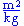xEncyclopedia
In electrical engineering
Electrical engineering
Electrical engineering is a field of engineering that generally deals with the study and application of electricity, electronics and electromagnetism. The field first became an identifiable occupation in the late nineteenth century after commercialization of the electric telegraph and electrical...

, the admittance (Y) is a measure of how easily a circuit or device will allow a current to flow. It is defined as the inverse
Multiplicative inverse
In mathematics, a multiplicative inverse or reciprocal for a number x, denoted by 1/x or x−1, is a number which when multiplied by x yields the multiplicative identity, 1. The multiplicative inverse of a fraction a/b is b/a. For the multiplicative inverse of a real number, divide 1 by the...

of the impedance
Electrical impedance
Electrical impedance, or simply impedance, is the measure of the opposition that an electrical circuit presents to the passage of a current when a voltage is applied. In quantitative terms, it is the complex ratio of the voltage to the current in an alternating current circuit...

(Z). The SI
Si
Si, si, or SI may refer to :- Measurement, mathematics and science :* International System of Units , the modern international standard version of the metric system...

unit of admittance is the siemens
Siemens (unit)
The siemens is the SI derived unit of electric conductance and electric admittance. Conductance and admittance are the reciprocals of resistance and impedance respectively, hence one siemens is equal to the reciprocal of one ohm, and is sometimes referred to as the mho. In English, the term...

(symbol S). Oliver Heaviside
Oliver Heaviside
Oliver Heaviside was a self-taught English electrical engineer, mathematician, and physicist who adapted complex numbers to the study of electrical circuits, invented mathematical techniques to the solution of differential equations , reformulated Maxwell's field equations in terms of electric and...

coined the term in December 1887.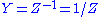where
Y is the admittance, measured in siemens
Siemens (unit)
The siemens is the SI derived unit of electric conductance and electric admittance. Conductance and admittance are the reciprocals of resistance and impedance respectively, hence one siemens is equal to the reciprocal of one ohm, and is sometimes referred to as the mho. In English, the term...

Z is the impedance, measured in ohms

Note that the synonymous unit mho, and the symbol ℧ (an upside-down uppercase omega Ω), are also in common use.

Resistance is a measure of the opposition of a circuit to the flow of a steady current, while impedance takes into account not only the resistance but also dynamic effects (known as reactance). Likewise, admittance is not only a measure of the ease with which a steady current can flow, but also the dynamic effects of the material's susceptance to polarization: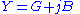where
•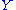is the admittance, measured in siemens (a.k.a. mho, the inverse of ohm).
•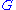is the conductance, measured in siemens.
•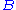is the susceptance
Susceptance
In electrical engineering, susceptance is the imaginary part of admittance. The inverse of admittance is impedance and the real part of admittance is conductance. In SI units, susceptance is measured in siemens...

, measured in siemens.
•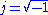## Conversion from impedance to admittance

The impedance, Z, is composed of real and imaginary parts,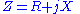where
R is the resistance, measured in ohms
X is the reactance, measured in ohms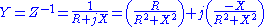Admittance, just like impedance, is a complex number, made up of a real
Real number
In mathematics, a real number is a value that represents a quantity along a continuum, such as -5 , 4/3 , 8.6 , √2 and π...

part (the conductance, G), and an imaginary
Imaginary number
An imaginary number is any number whose square is a real number less than zero. When any real number is squared, the result is never negative, but the square of an imaginary number is always negative...

part (the susceptance, B), thus:,

where G (conductance) and B (susceptance) are given by: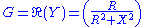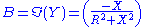The magnitude and phase of the admittance are given by: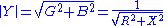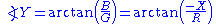where
G is the conductance, measured in siemens
Siemens (unit)
The siemens is the SI derived unit of electric conductance and electric admittance. Conductance and admittance are the reciprocals of resistance and impedance respectively, hence one siemens is equal to the reciprocal of one ohm, and is sometimes referred to as the mho. In English, the term...

B is the susceptance, also measured in siemens
Siemens (unit)
The siemens is the SI derived unit of electric conductance and electric admittance. Conductance and admittance are the reciprocals of resistance and impedance respectively, hence one siemens is equal to the reciprocal of one ohm, and is sometimes referred to as the mho. In English, the term...

Note that (as shown above) the signs of reactances become reversed in the admittance domain, i.e. capacitive susceptance is positive and inductive suceptance is negative.

In mechanical systems (particularly in the field of haptics), an admittance is a dynamic mapping from force to motion. In other words, an equation (or virtual environment) describing an admittance would have inputs of force and would have outputs such as position or velocity. So, an admittance device would sense the input force and "admit" a certain amount of motion.

Similar to the electrical meanings of admittance and impedance, an impedance in the mechanical sense can be thought of as the "inverse" of admittance. That is, it is a dynamic mapping from motion to force. An impedance device would sense the input motion and "impede" the motion with some force.

An example of these concepts is a virtual spring. The equation describing a spring is Hooke's Law
Hooke's law
In mechanics, and physics, Hooke's law of elasticity is an approximation that states that the extension of a spring is in direct proportion with the load applied to it. Many materials obey this law as long as the load does not exceed the material's elastic limit. Materials for which Hooke's law...

,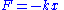If the input to the virtual spring is the spring displacement, x, and the output is the force that the virtual spring applies, F, then the virtual spring would be classified as an impedance.
If the input to the virtual spring is the force applied to the spring, F, and the output is the spring displacement, x, then the virtual spring would be classified as an admittance.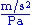or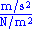or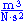or, in primary units